# Requiring an amyloid-β1-42 biomarker may improve the efficiency of a study, and simulations may help in planning studies

## Abstract

A recent article by Schneider and colleagues has generated a lot of interest in simulation studies as a way to improve study design. The study also illustrates the foremost principal in simulation studies, which is that the results of a simulation are an embodiment of the assumptions that went into it. This simulation study assumes that the effect size is proportional to the mean to standard deviation ratio of the Alzheimer Disease Assessment Scale - cognitive subscale in the population being studied. Under this assumption, selecting a subgroup for a clinical trial based on biomarkers will not affect the efficiency of the study, despite achieving the desired increase in the mean to standard deviation ratio.

## Simulation study

The simulation study reported by Schneider and colleagues is a valuable contribution to the field of Alzheimer's disease. The study was conducted under a detailed protocol and clearly lays out the assumptions that were made and the criteria that were used for each set of simulations . The article makes the point that some situations are complicated enough that standard power calculations do not capture the whole picture, because they require simplifying assumptions that may not hold. In these cases, power calculations may more accurately reflect reality when based on simulations that do not rely as much on distributional assumptions.

In this study, one critical assumption is the basis of the main conclusion. Table 1 presents the results taken from Schneider and colleagues' study, including the mean and standard deviation (SD) for each group for the Alzheimer Disease Assessment Scale - cognitive subscale (ADAS-cog).

The difference between group means divided by the SD is called a standardised difference (or Cohen's D value ) and allows estimation of power based on a t test. If you take the placebo group mean and subtract the treatment group mean and then divide that difference by the placebo SD, using numbers that are all shown in the table, you obtain the effect size shown in the third column, within the rounding error (25%, 35% and 45%). This exercise illustrates that the effect size used in this simulation study increases and decreases proportionally to the standardised difference, which is tied mathematically to the power. In other words, although the sensitivity of the ADAS-cog to decline over time is increased with the biomarker selection methods, the treatment difference was decreased in order to maintain the same standardised difference.

Although this same type of approach seems to have been taken for the Clinical Dementia Rating scale sum of boxes (CDR-sb), calculating the observed effect size (Cohen's D value) by taking the difference between group means divided by the SD does not correspond to the planned effect size shown in Schneider and colleagues' Table 3 . The rows with a planned effect size of 25% have calculated values ranging from 21 to 22%, the rows with a planned effect size of 35% have calculated values ranging from 27 to 30%, and the rows with a planned effect size of 45% have calculated values ranging from 35 to 39% (data not shown). It is unclear why the simulations consistently provide outcomes with lower effect sizes than those planned, particularly when the ADAS-cog observed effect sizes are not biased.

## Defining effect size

There are several different ways to define effect size [3, 4]. Because the estimated power is often compared between different scenarios assuming an equal effect size, it is important to know which effect size is assumed to be equal, and what impact that assumption is expected to have on the estimated power. The means and SDs referred to here are the mean and SD of the change from baseline for each treatment group.

Four different definitions of effect size will be compared: one that is unstandardised (the absolute difference); and three that are standardised values, calculated through dividing by some type of scaling factor (Cohen's D value using the baseline SD, Cohen's D value using the change from baseline SD, and the percentage of placebo decline that uses the placebo mean change from baseline).

## Absolute difference

The absolute difference between treatment groups is calculated by simply subtracting the two treatment group means (usually the mean changes from baseline):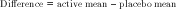This observed treatment difference is often reported in addition to some type of standardised effect size. This difference is nonstandardised so it is difficult to compare between different instruments, because a 2-point difference on the ADAS-cog is not comparable with a 2- point difference on the CDR-sb. The absolute difference on a single scale is also difficult to compare between studies if the studies include different patient populations. For instance, a 2-point difference on the ADAS-cog may be more meaningful in a mild patient population than in a moderate one. Because of these issues, a standardised treatment effect is often reported in addition to the absolute treatment difference.

## Cohen's D value using the baseline standard deviation

One way to standardise the effect size is to divide the observed treatment difference by the baseline SD. This procedure is common and appropriate when the baseline scores represent some type of normal or healthy state from which patients may deteriorate, and then to which they may possibly return. This value is the number of SDs of difference between the two groups relative to the baseline population:In the case of Alzheimer's disease, mild cognitive impairment or prodromal Alzheimer's disease, the base-line population represents an already deteriorated patient population so standardising based on this non-healthy population can therefore lead to unusual effect sizes. For instance, a homogeneous group of patients - that is, a population with very similar severity at baseline - may have a SD that is one-half that of a less homogeneous population with the same baseline mean. If the same absolute treatment difference is observed in these two populations, then the first population would have a Cohen's D value that is twice as large as the second population due solely to the differences in baseline variability.

## Cohen's D value using change from baseline standard deviation (z-score effect size or standardised difference)

If the absolute treatment difference is divided by the SD of the change from baseline, then this effect size also represents the number of SDs of difference between the two groups relative to the changes from baseline that were observed. This is a type of z-score calculation and is often referred to as a standardised difference. This is the effect size that was used by Schneider and colleagues :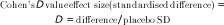Although the placebo SD is shown in the equation, this calculation sometimes uses the pooled SD across treatment groups.

This type of effect size calculation is less susceptible to population differences at baseline, but it is still susceptible to differences in the homogeneity of the change over time. So if a group is more homogeneous at baseline, it is also likely that the changes from baseline will be more homogeneous, making comparison between the groups complicated.

The other issue that factors into this calculation is the sensitivity of the instrument. If an instrument is used that has substantial variability in the change from baseline over time, then the Cohen's D values will be lower than with an instrument with less variability. Although one could argue that an effect on a more variable instrument should be penalised because of the variability, it means that a 35% effect, for instance, on a variable instrument could be quite a lot larger than a 35% effect on a less variable instrument. There is a direct relationship between this standardised difference (D), the sample size and power for a two-sample t test: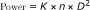where K is a constant that depends on α (the type 1 error rate, traditionally selected to be 0.05), and n is the sample size per group.

## Percentage placebo effect

Because Alzheimer's disease, including mild cognitive impairment and prodromal Alzheimer's disease, is a degenerative disease, a natural scaling factor is the placebo rate. Dividing the absolute difference by the placebo mean change from baseline results in an effect size that represents the percentage reduction in the placebo decline - an effect size >100% indicates an improvement over baseline: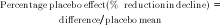This effect size has the advantage that it is standardised to time rather than to the variability of a group of patients. A 30% effect size, for instance, can therefore be interpreted as a reduction of 30% in the rate of the placebo group. This effect size is easily comparable across different instruments in the same disease state because the sensitivity of the instrument does not affect the effect size. This effect size is also at least somewhat comparable between patient groups in different disease states, since any floor or ceiling effects that may impact the instrument sensitivity may similarly affect the difference, thus not impacting the effect size.

An additional metric, referred to as the signal to noise ratio, measures the sensitivity of a particular instrument in a specific population of patients and is useful when using the percentage placebo effect: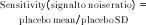This metric allows comparison of instruments within a population, and also allows estimation of ceiling and floor effects. The signal to noise ratio multiplied by the percentage placebo effect is equal to the Cohen's D value effect size using the change from baseline SD. This relationship allows us to make a set of power curves based on the sensitivity of an instrument which can then be used to compare the power between different percentage placebo effects.

## Discussion

The three farthest right columns in Table 1 show that as the sensitivity increases, the percentage placebo effect size decreases. Consider the example shown in the first three rows of Table 1 with n = 100 per group and a dropout rate of 20%. The sensitivity of the ADAS-cog increases from 0.51 for the amnestic mild cognitive impairment (aMCI) group to 0.62 or 0.63 with the bio-marker selected groups. If the percentage placebo effect of 0.69 that is shown for the aMCI group is also used for the two biomarker selected groups, we can estimate the power using Figure 1 and 2. For the aMCI group, the power would be approximately 0.60 (using Figure 1 effect size = 0.70; PASS 2005  used for all power calculations). For the biomarker selected groups, the power is approximately 0.75 (using Figure 2 effect size = 0.70). Also using Figure 2 a sample size of approximately 70 per group can achieve power of 0.60, comparable with the power achieved with a sample size of 100 in the aMCI group.

There are critical differences in the approaches that have been used to discuss power and effect size in clinical trials. Below are three assumptions that correspond to assuming equal effects using three different methods of reporting treatment effects.

The first method is the absolute difference. Assuming that the absolute treatment difference (point difference) is the same across different trial scenarios implies that a treatment can give X points benefit no matter how much the placebo group declines, how sensitive the instrument, or how heterogeneous the population being studied. This approach cannot reasonably be used to compare power between two different instruments such as the ADAS-cog and CDR-sb, since the same point differences on these two instruments would not be comparable.

The second reporting method is the standardised difference or Cohen's D value using the placebo standard deviation (used in Schneider and colleagues' article ). Assuming that the standardised difference is the same across trial scenarios implies that a treatment gives the same percentage benefit relative to the SD of the change from baseline of the instrument. If different instruments used to measure a disease are similarly sensitive to decline over time, then this type of comparison may be valid. Using this method, however, an increase in measurement error, such as that introduced with careless instrument administration, would be associated with an increase in the expected efficacy of the treatment under consideration, sufficient to counteract the decrease in power due to increased variability.

The final method is the percentage placebo effect. Assuming that the percentage difference relative to placebo is the same across trial scenarios implies that a treatment gives the same percentage benefit relative to the decline of the placebo group. This approach could only be considered for a disease with an increasing outcome or a degenerative disease such as Alzheimer's disease. In Alzheimer's disease, use of the method assumes that the treatment is expected to reduce the decline by the same percentage across different trial scenarios. This assumption may be justified when studying the same patient population with different instruments; it may not be reasonable when comparing different disease stages, however, since a treatment may not have the same percentage benefit in these different patient populations. This is the basis of the argument for earlier treatment. Treatments may be able to affect the disease more in the earlier stages. It is not clear whether this would be related to the position in the disease or to the slower decline rate that may be expected earlier in the disease (which, incidentally, may be due to a ceiling effect of an instrument). When selecting a population based on biomarkers in order to increase the decline rate seen over the study period, it is not clear whether the same percentage effect would be expected in this subgroup, or whether the percentage effect might actually go down due to the more rapid progression of this subgroup. This method does have the advantage of not depending on the sensitivity of the instrument being used.

Figure 3 shows the difference between a percentage placebo effect and a standardised difference. Figure 3a shows a 50% effect as a percentage of the placebo decline of 4 points (2-point effect). Figure 3b shows a 50% standardised difference effect when the SD is 6 points (3-point effect). Using the same 50% effect but a scenario with a smaller placebo decline (3-point decline instead of 4-point decline), a 1.5-point difference is obtained for the placebo decline effect(Figure 3c) and a 3-point difference for the standardised effect(Figure 3d) since the SD was kept at 6 points. These data illustrate the difference between a percentage effect relative to placebo and a standardised difference that is relative to the SD.

Although observing similar power when comparing biomarker selected groups and the aMCI group as a whole is a direct result of using the same standardised difference, column J in Table 1 shows that the absolute difference is also quite similar between the biomarker selected groups and the aMCI group, and is actually larger for the aMCI group. This indicates the conclusion that the power may not be much improved with biomarker selection may be valid if a treatment has a similar absolute difference in all three groups. In fact, the power differences would be even less than were shown since the absolute treatment difference shown for the aMCI group is slightly smaller than for the two biomarker selected groups.

The question therefore comes down to the issue of whether selecting a faster declining patient group, which generally increases the mean to SD ratio of an instrument (by increasing both the mean and the SD, but increasing the mean more than the SD), will also result in an increase in effect proportional to the increase in the mean placebo decline. Previous power comparisons have assumed that it will. Schneider and colleagues assume that this selection would not but that the effect will stay proportionally the same relative to the SD, resulting in no effect on power . Another way of assuming that this selection will not result in an increase in effect proportional to the increase in the mean placebo decline would be to assume a constant absolute treatment effect. This assumption also results in very similar power between aMCI and biomarker selected groups.

## Conclusions

Simulation studies are an appropriate way to explore the impact of different study design decisions in order to improve the study design. The results of a simulation are an embodiment of the assumptions that went into it. This simulation study assumes that the effect size is proportional to the mean to SD ratio of the ADAS-cog in the population being studied. Because this type of effect size increases proportionally to the mean to SD ratio, increasing the mean to SD ratio cannot affect the power. The small differences in power that were observed by Schneider and colleagues between the three selection methods are probably due to the differences in simulating the measurement error component of the treatment response. In addition, the CDR-sb is not able to show increased power despite its improved sensitivity to decline (signal to noise ratio), because the effect size, as defined, increases proportionally to the signal to noise ratio - although there are some concerns about the observed effect sizes calculated from Table 3 in Schneider and colleagues' paper . Assuming a constant absolute treatment effect also results in very similar estimated power between the aMCI and biomarker selected groups.

Assuming a constant percentage placebo effect size does show differences in the power for the selected patient subgroups. This assumption also shows improved power of the CDR-sb over the ADAS-cog, specifically due to the improvement in sensitivity or signal to noise ratio.

Separating the evaluation of an instrument in its ability to measure the decline in Alzheimer's disease over time (sensitivity) from the ability of a treatment to affect the decline over time (percentage placebo effect size) clarifies the discussion of power, efficiency and sample size.

There is no way to know whether selecting a faster declining patient group, which generally increases the mean to SD ratio of an instrument, will also result in an increase in effect proportional to the increase in the mean placebo decline. Previous power comparisons have assumed that it will. Both the approach described in Schneider and colleagues' article and an approach using a constant absolute treatment effect assume that this selection would not increase the effect, resulting in very similar power between the aMCI and biomarker selected groups.

## Abbreviations

Alzheimer Disease Assessment Scale - cognitive subscale

aMCI:

amnestic mild cognitive impairment

CDR-sb:

Clinical Dementia Rating scale sum of boxes

SD:

standard deviation.

## References

1. Schneider LS, Kennedy RE, Cutter GR, Alzheimer's Disease Neuroimaging Initiative: Requiring an amyloid-β1-42 biomarker for prodromal Alzheimer's disease or mild cognitive impairment does not lead to more efficient clinical trials. Alzheimers Dement. 2010, 6: 367-377. 10.1016/j.jalz.2010.07.004.

2. Cohen J: Statistical Power for the Behavioral Sciences. 1969, New York: Academic Press

3. Ellis P: The Essential Guide to Effect Sizes: Statistical Power, Meta-Analysis, and the Interpretation of Research Results. 2010, Cambridge: Cambridge University Press

4. Snyder PA, Lawson S: Evaluating results using corrected and uncorrected effect size estimates. J Exp Educ. 1993, 61: 334-349.

5. PASS 2005. [http://www.ncss.com]

## Author information

Authors

### Corresponding author

Correspondence to Suzanne B Hendrix.

### Competing interests

The author declares that they have no competing interests.

## Authors’ original submitted files for images

Below are the links to the authors’ original submitted files for images.

## Rights and permissions

Reprints and Permissions

Hendrix, S.B. Requiring an amyloid-β1-42 biomarker may improve the efficiency of a study, and simulations may help in planning studies. Alz Res Therapy 3, 10 (2011). https://doi.org/10.1186/alzrt69